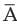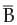# Test: Probability And Expected Value By Mathematical Expectation- 2

## 40 Questions MCQ Test Quantitative Aptitude for CA CPT | Test: Probability And Expected Value By Mathematical Expectation- 2

Description
Attempt Test: Probability And Expected Value By Mathematical Expectation- 2 | 40 questions in 40 minutes | Mock test for CA Foundation preparation | Free important questions MCQ to study Quantitative Aptitude for CA CPT for CA Foundation Exam | Download free PDF with solutions
QUESTION: 1

Solution:
QUESTION: 2

Solution:
QUESTION: 3

### f(x), the probability mass function of a random variable x satisfies

Solution:
QUESTION: 4

Variance of a random variable x is given by

Solution:
QUESTION: 5

If two random variables x and y are related by y = 2 – 3x, then the SD of y is given by

Solution:
QUESTION: 6

Probability of getting a head when two unbiased coins are tossed simultaneously is

Solution:
QUESTION: 7

If an unbiased coin is tossed twice, the probability of obtaining at least one tail is

Solution:
QUESTION: 8

If an unbiased die is rolled once, the odds in favour of getting a point which is a multiple of 3 is

Solution:

Total number =6
Getting a 'multiple of 3' = 2 . So, probability = 2/6 =1/3

QUESTION: 9

A bag contains 15 one rupee coins, 25 two rupee coins and 10 five rupee coins. If a coin is selected at random from the bag, then the probability of not selecting a one rupee coin is

Solution:
QUESTION: 10

A, B, C are three mutually independent with probabilities 0.3, 0.2 and 0.4 respectively.What is P (A ∩ B ∩ C)?

Solution:
QUESTION: 11

If two letters are taken at random from the word HOME, what is the Probability that none of the letters would be vowels?

Solution:
QUESTION: 12

If a card is drawn at random from a pack of 52 cards, what is the chance of getting a Spade or an ace?

Solution:
QUESTION: 13

If x and y are random variables having expected values as 4.5 and 2.5 respectively, then the expected value of (x–y) is

Solution:
QUESTION: 14

If variance of a random variable x is 23, then what is the variance of 2x+10?

Solution:
QUESTION: 15

What is the probability of having at least one ‘six’ from 3 throws of a perfect die?

Solution:
QUESTION: 16

(Direction 16 - 38) Write down the correct answers. Each question carries 2 marks.

Q.Two balls are drawn from a bag containing 5 white and 7 black balls at random. What is the probability that they would be of different colours?

Solution:
QUESTION: 17

What is the chance of throwing at least 7 in a single cast with 2 dice?

Solution:
QUESTION: 18

What is the chance of getting at least one defective item if 3 items are drawn randomly from a lot containing 6 items of which 2 are defective item?

Solution:
QUESTION: 19

If two unbiased dice are rolled together, what is the probability of getting no difference of points?

Solution:
QUESTION: 20

If A, B and C are mutually exclusive independent and exhaustive events then what is the probability that they occur simultaneously?

Solution:
QUESTION: 21

There are 10 balls numbered from 1 to 10 in a box.if one ofthem is selected at random whatisthe probability that the number printed onthe ball woukd be an odd number greater than 4

Solution:
QUESTION: 22

Following are the wages of 8 workers in rupees:

50, 62, 40, 70, 45, 56, 32, 45

If one of the workers is selected at random, what is the probability that his wage would be lower than the average wage?

Solution:
QUESTION: 23

A, B and C are three mutually exclusive and exhaustive events such that P (A) = 2 P (B) = 3P(C). What is P (B)?

Solution:
QUESTION: 24

For two events A and B, P (B) = 0.3, P (A but not B) = 0.4 and P (not A) = 0.6. The events A and B are

Solution:
QUESTION: 25

A bag contains 12 balls which are numbered from 1 to 12. If a ball is selected at random, what is the probability that the number of the ball will be a multiple of 5 or 6 ?

Solution:
QUESTION: 26

Given that for two events A and B, P(A)=3/5, P(B)=2/3 and P(A union B) =3/4, what is P(A|B)?

Solution:

P(A|B) = P(A&B)/P(B)
So first we have to find P(A&B)
P(AUB) = P(A) + P(B) - P(A&B)3/4 = 3/5 + 2/3 - P(A&B)
Multiply through by 60
45 = 36 + 40 - 60P(A&B)
45 = 76 - 60P(A&B)
-31 = -60P(A&B)
31/60 = P(A&B)
Go back to
P(A|B) = P(A&B)/P(B)
P(A|B) = (31/60)/(2/3)
P(A|B) = (31/60)(3/2)
P(A|B) = 31/40 = 0.775

QUESTION: 27

For two independent events A and B, what is P (A+B), given P(A) = 3/5 and P(B) = 2/3?

Solution:

P(A + B) = 13/15
Step-by-step explanation:
P(A) = Probability of Event A Heppening
P(A') = Probability of Event A not Happeining
P(A) = 3/5  => P(A') = 1 - 3/5  = 2/5
P(B) = 2/3  => P(B') = 1 - 2/3  = 1/3
P(A + B) = 1 - Probability of Event A & B not happening
P(A + B) = 1  - P(A')P(B')
=> P(A + B) = 1  -  (2/5)(1/3)
=> P(A + B) = 1  -  2/15
=> P(A + B) = 13/15

QUESTION: 28

If P (A) = p and P (B) = q, then

Solution:
QUESTION: 29

​If P (∪ B ) = 5/6, P(A) = ½ and P () = 2/3, , what is P (A ∪ B) ?

Solution:

P(A u B ) = P(A) + P(B) - P(A Π B )

5/6 = 1/2 + 2/3 - P ( A Π B )

5/6 - 7/6 = - P ( A Π B )

-2/6 = - P( A Π B )

P ( A Π B ) = 1/3

QUESTION: 30

If for two independent events A and B, P (A ∪ B) = 2/3 and P (A) = 2/5, what is P (B)?

Solution:
QUESTION: 31

If P (A) = 2/3, P (B) =3/4, P (A/B) = 2/3, then what is P (B / A)?

Solution:
QUESTION: 32

If P (A) = a, P (B) = b and P (P (A ∩ B) = c then the expression of P (A’ ∩ B’) in terms of a, b and c is

Solution:
QUESTION: 33

For three events A, B and C, the probability that only A occur is

Solution:
QUESTION: 34

It is given that a family of 2 children has a girl, what is the probability that the other child is also a girl ?

Solution:
QUESTION: 35

Two coins are tossed simultaneously. What is the probability that the second coin would show a tail given that the first coin has shown a head?

Solution:
QUESTION: 36

If a random variable x assumes the values 0, 1 and 2 with probabilities 0.30, 0.50 and 0.20, then its expected value is

Solution:
QUESTION: 37

If two random variables x and y are related as y = –3x + 4 and standard deviation of x is 2, then the standard deviation of y is

Solution:
QUESTION: 38

If 2x + 3y + 4 = 0 and v(x) = 6 then v (y) is

Solution:
QUESTION: 39

(Direction 39 - 40) Write down the correct answers. Each question carries 5 marks.

Q. What is the probability that a leap year selected at random would contain 53 Saturdays?

Solution:
QUESTION: 40

If an unbiased coin is tossed three times, what is the probability of getting more that one head?

Solution:Use Code STAYHOME200 and get INR 200 additional OFF Use Coupon Code